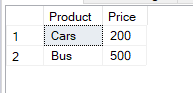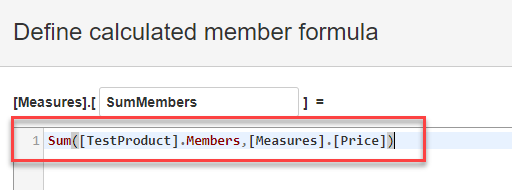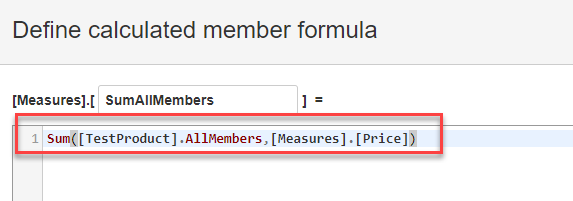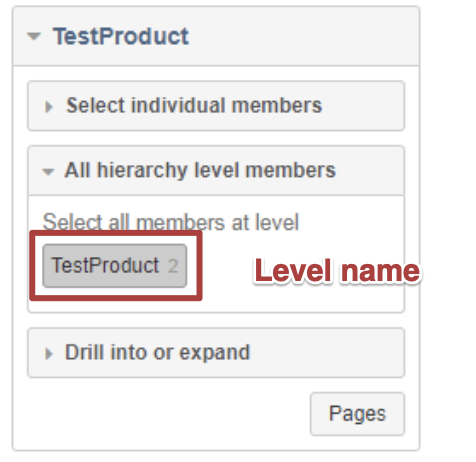# How to calculate Total Sum of Rows as a measure

I’m using the SQL Application to fetch data into EazyBI. What I want to calculate is a measure that will give me the total of all the rows in that report.

For example, I have created a test cube with this data.And the report is created like this.

Now I’ve created two measures like the following:

1.2.Including these two calculated measures in the report.

I’m expecting the measures will return 700 as it is the total of all the members in the dimension, however, the counts appear elevated.

Am I missing anything here? If yes, can you pls guide me on how to obtain the 700 for each of the records in a similar fashion?

Hi @aveek.das ,

Cool, you have imported and mapped data and almost there with the calculation!
The thing is, functions `Members` and `AllMembers` retrieve all dimension members, not only the lowest members as products, therefore, the sum over all dimension members is larger than the expected sum of products.

A bit of theory
In eazyBI, all dimensions are hierarchical. It means, dimensions contain not only members that are particular items (as products in your case), but also members that aggregates all items or, in more complex dimensions, also subgroups of those members; it depends how many levels the hierarchy has. Dimensions with simple hierarchies as yours have members in two levels: particular members for each product and a default member or All level member that aggregates the products. More about dimensions and members: https://docs.eazybi.com/display/EAZYBI/Main+concepts#Mainconcepts-Dimensions (and the following section).

What went wrong?

When you use `[TestProduct].Members`, it retrieves values from all dimension standard members (i.e., products and the default member). In your case, members Cars, Bus, and All Products are retrieved and all their values (500+200+700) are summed.
When `[TestProduct].AllMembers` is used, also calculate members of this dimension are retrieved and the sum is calculated over this larger set (I do not see them in your screenshots, as calculates members are in “Select individual members” sections).

What is the solution?
In your case, you may want to use the value of the Product dimension default member itself as it already contains all products. If you want to display the total value in rows against each product, you may create a measure with a tuple that overrides the price of the product with total value:

``````([Measures].[Price],
[TestProduct].DefaultMember)
``````

`[TestProduct].[TestProduct].Members`